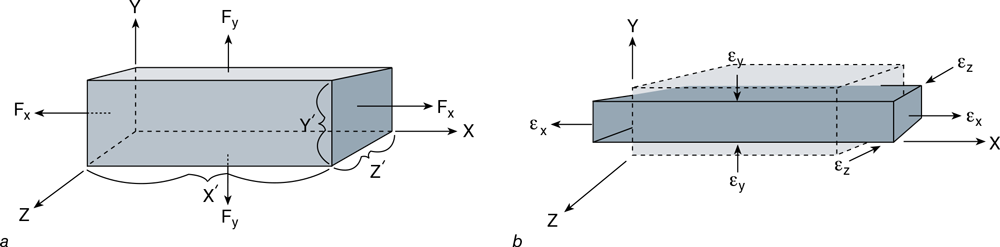Feedback

### This is an excerpt from Biomechanics of Injury-3rd Edition by Ronald F. Zernicke,Steven Broglio & William C. Whiting.

We have so far considered only the ­simple case of uniaxial loading. In most real-­life situations, however, the forces applied to a body are multidimensional, and hence an understanding of multiaxial loading and its effects is essential. An analy­sis of multiaxial loading uses the same stress and strain concepts just discussed and extends them into two-­ and three-­dimensional space. Although the biaxial and triaxial responses are illustrated for tensile loading only, the concepts are equally applicable to compressive loading and to force vectors with reversed orientation. The following formulations are for linearly elastic materials.FIGURE 3.29 Biaxial loading. (a) Applied forces in the X and Y direction (Fx and Fy, respectively) load the material biaxially. (b) Elongation caused by Fx and the resulting perpendicular contraction in the Y and Z directions. Dashed lines indicate conformation prior to loading. Solid lines indicate conformation while loaded.

Biaxial (Two-­Dimensional) Loading Responses Consider a three-­dimensional body (figure 3.29) with sides of length X′, Y′, and Z′ that is subjected to axial forces Fx and Fy. The stresses produced in the X and Y directions are as follows:The X-­direction stress (σx) ­will, according to Poisson’s effect, cause deformation in all three directions. Elongation in the X direction and contraction in the Y and Z directions are shown in figure 3.29b. By applying equations 3.34 and 3.35, we obtain X-­ and Y-­direction strains attributable to σx:We similarly obtain Y-­ and X-­direction strains attributable to σy:To obtain the combined effect of σx and σy, we add the strain effects just calculated. The net strains in the X and Y directions are thenWe have presented two normal stresses, σx and σy. As previously described, a tangential, or shear, stress (τ) is also created. In the case of biaxial loading, shear stress (τxy) is created as shown in figure 3.30a.